# Data Science Pipeline: Financial Data and Machine Learning¶

CMSC320 Final Project

Name: Ryan Downing

Due: May 18, 2020

# Introduction¶

In the past few decades, the world of finance has been transformed by data. Now more than ever, companies are utilizing big data in attempt to predict the future. More specifically in regards to the stock market, investors want to predict whether a stock's share price will appreciate, or depreciate in the future.

This tutorial will walkthrough the data science process using data from: https://www.quandl.com/tables/SF1/SHARADAR-SF1

We will explore how we can use visualizations to help investors understand market conditions, and then apply more advanced techniques of machine learning to predict future performance. In particular, this tutorial will compare the prediction performance of a multivariate logistic regression against a random forest prediction.

In :
import datetime
import requests
import psycopg2
import numpy as np
import pandas as pd
import seaborn as sns
import matplotlib.pyplot as plt

SEED = 1234
np.random.seed(SEED)
CONNECTION_PARAMS = {
'database': ## REDACTED ##
'user': ##################
'host': ##################
'port': #### REDACTED ####
}
conn = psycopg2.connect(**CONNECTION_PARAMS)
cur = conn.cursor()


# Data Curation¶

## Aggregation¶

The data used in this tutorial comes from a SQL database as defined above. To concentrate the scope of this analysis, we will be looking at those companies that are currently in the S&P500 (taken from wikipedia). In addition, we will be looking at data between January 1st, 2005, and December 31st 2019. This gives us 15 years of data to work with, of which 12 years (2005-2017) will be used for training the model, and 3 years (2017-2020) will be reserved to test the model.

Each entry in the database represents a SEC 10Q filing for a company, given by ticker. The attributes represent the different data elements of the SEC filing. For this analysis, we will focus on those given by the income statement, statement of cash flows, and balance sheet. We will also look at many of the common metrics used by investors to asses companies. A more thorough documentation of these attributes can be found here.

The code below constructs a SQL query to pull the necessary data from the database. To do this we must first pull the table of S&P companies from the wikipedia page, and then select the Symbol column which gives us the ticker of each company. We must then create a list of all the attributes we want to pull from the database, features.

Since we are interested in the change of share price for a given ticker over time, I also add datekey, ticker, and price to the attributes list. We can then build a string query for the SQL database by joining the ticker conditions with OR and the datae conditions with AND, and then finally combine both of these with AND for the WHERE clause of the query.

The database also needs to know the list of parameters these conditions refer to, so we can simply build a list by combining the tickers with the start and end date. Now we can create the full query using the SELECT, FROM, WHERE template.

note: dimension = \'ARQ\' refers to "as-reported quarterly" which avoids future-bias, more information can be found here

In :
table = 'fundamental_raw' # table name in database
url = "https://en.wikipedia.org/wiki/List_of_S%26P_500_companies"
sp_tickers = pd.read_html(url)['Symbol'].unique()  # Gets table from wikipedia and selects unique tickers
start_date = datetime.datetime(year=2005, month=1, day=1)
end_date = datetime.datetime(year=2019, month=12, day=31)

# Income statement
IS = ['revenue', 'cor', 'sgna', 'rnd', 'opex',
'intexp', 'taxexp', 'ebit', 'netinc', 'eps']

# Statement of Cash flows
CF = ['capex', 'ncfbus', 'ncfinv', 'ncff',
'ncfdebt', 'ncfcommon', 'ncfdiv', 'ncfi',
'ncfo', 'ncfx', 'ncf', 'sbcomp', 'depamor']

# Balance Sheet
BS = ['assets', 'cashneq', 'investments', 'investmentsc',
'investmentsnc', 'deferredrev', 'deposits','ppnenet',
'inventory', 'taxassets', 'receivables', 'payables',
'intangibles', 'liabilities', 'equity', 'retearn',
'assetsc', 'assetsnc', 'liabilitiesc', 'liabilitiesnc',
'taxliabilities', 'debt', 'debtc', 'debtnc']

# Common Metrics
MT = ['marketcap', 'ev', 'fcf', 'gp', 'opinc', 'grossmargin',
'netmargin', 'ebitdamargin', 'payoutratio', 'evebitda',
'evebit', 'pe', 'sps', 'ps', 'pb', 'de', 'divyield',
'currentratio', 'workingcapital', 'fcfps', 'bvps']

features = IS + CF + BS + MT  # create a single list of all the features we are interested in

attributes = ['datekey', 'ticker', 'price'] + features  # Create list of all attributes
ticker_conds = ' OR '.join(['ticker = %s' for ticker in sp_tickers])  # query for each ticker
date_conds = 'datekey >= %s AND datekey <= %s'  # date query
query_conds = f'({ticker_conds}) AND ({date_conds}) AND (dimension = \'ARQ\')'  # combine to create full "WHERE" query
attrs_query = ', '.join(attributes)
params = list(sp_tickers) + [start_date, end_date]  # create list of parameters for SQL query

fundamental_query = f'SELECT {attrs_query} FROM {table} WHERE {query_conds}'  # create full query


Now that we have the full query constructed, we can execute the query in the database using cur.execute. The response gives us the full dataset, which we can simply put into a pandas dataframe, using the column names received from cur.description.

In :
# Execute query and store into dataframe
cur.execute(fundamental_query, params)
reponse = cur.fetchall()
col_names = [desc.name for desc in cur.description]
fund = pd.DataFrame(data=reponse, columns=col_names)

Out:
datekey ticker price revenue cor sgna rnd opex intexp taxexp ... pe sps ps pb de divyield currentratio workingcapital fcfps bvps
0 2005-03-10 A 23.91 1.658000e+09 907000000.0 424000000.0 232000000.0 656000000.0 0.0 21000000.0 ... 30.823 3.377 1.632 3.117 0.898 0.0 2.625 2.878000e+09 0.204 7.674
1 2005-06-06 A 24.52 1.688000e+09 898000000.0 460000000.0 248000000.0 708000000.0 0.0 25000000.0 ... 32.387 3.438 1.708 3.114 0.879 0.0 2.727 3.048000e+09 0.405 7.880
2 2005-09-07 A 32.49 1.688000e+09 923000000.0 432000000.0 244000000.0 676000000.0 0.0 25000000.0 ... 42.702 3.417 2.342 4.067 0.862 0.0 2.781 3.155000e+09 0.174 7.992
3 2006-01-17 A 33.76 1.050000e+08 -111000000.0 287000000.0 14000000.0 301000000.0 0.0 84000000.0 ... 44.266 0.210 2.817 3.547 0.654 0.0 2.297 2.511000e+09 0.858 8.162
4 2006-03-09 A 36.21 1.336000e+09 657000000.0 445000000.0 189000000.0 634000000.0 0.0 15000000.0 ... 5.160 2.825 3.257 3.751 0.919 0.0 2.826 2.989000e+09 -0.372 8.841

5 rows × 71 columns

## Cleaning¶

To get this data into a usable format for analysis, we must do some cleaning to prepare it. Fortunately, this database is already in a fairly clean format. However, we must convert the datekey column to datetime datatype, and then rename it date. Now that we have this, we can easily extract the year using dt.year to create a year column.

Since companies have the ability to post corrections for their SEC filing, there is a chance that a company has multiple reports for a single date. To correct for this, we should drop duplicate rows, regarding ticker and date.

In :
fund['datekey'] = pd.to_datetime(fund['datekey'])
fund.rename(columns={'datekey': 'date'}, inplace=True)
fund['year'] = fund['date'].dt.year

fund.drop_duplicates(subset=['date', 'ticker'], keep='last', inplace=True)

Out:
date ticker price revenue cor sgna rnd opex intexp taxexp ... sps ps pb de divyield currentratio workingcapital fcfps bvps year
0 2005-03-10 A 23.91 1.658000e+09 907000000.0 424000000.0 232000000.0 656000000.0 0.0 21000000.0 ... 3.377 1.632 3.117 0.898 0.0 2.625 2.878000e+09 0.204 7.674 2005
1 2005-06-06 A 24.52 1.688000e+09 898000000.0 460000000.0 248000000.0 708000000.0 0.0 25000000.0 ... 3.438 1.708 3.114 0.879 0.0 2.727 3.048000e+09 0.405 7.880 2005
2 2005-09-07 A 32.49 1.688000e+09 923000000.0 432000000.0 244000000.0 676000000.0 0.0 25000000.0 ... 3.417 2.342 4.067 0.862 0.0 2.781 3.155000e+09 0.174 7.992 2005
3 2006-01-17 A 33.76 1.050000e+08 -111000000.0 287000000.0 14000000.0 301000000.0 0.0 84000000.0 ... 0.210 2.817 3.547 0.654 0.0 2.297 2.511000e+09 0.858 8.162 2006
4 2006-03-09 A 36.21 1.336000e+09 657000000.0 445000000.0 189000000.0 634000000.0 0.0 15000000.0 ... 2.825 3.257 3.751 0.919 0.0 2.826 2.989000e+09 -0.372 8.841 2006

5 rows × 72 columns

Since we are not concerned with the share price, but rather the change in share price, we must calculate the percent change in price between report periods for each ticker. To do this we must first ensure the data is sorted by date so the percent change is calculated between consecutive periods. Then we can just groupby ticker and use pandas pct_change() method to calculate the price change for each ticker.

Calculating change results in a NaN in the first row for each ticker, so we should drop these rows. Then we transform the price_change to a binary up or down signal using np.where.

In :
fund.sort_values('date', inplace=True)
fund['price_change'] = fund.groupby('ticker')['price'].pct_change()
fund.dropna(subset=['date', 'ticker', 'price', 'price_change'], inplace=True)
fund['movement'] = np.where(fund['price_change'] >= 0, 'up', 'down')

Out:
date ticker price revenue cor sgna rnd opex intexp taxexp ... pb de divyield currentratio workingcapital fcfps bvps year price_change movement
23902 2005-03-04 CI 30.057 4.342000e+09 2.198000e+09 1.331000e+09 0.0 1.331000e+09 0.0 253000000.0 ... 2.280 14.579 0.004 NaN NaN 0.631 13.183 2005 0.005251 up
8303 2005-03-10 SNPS 18.020 2.413040e+08 6.997500e+07 1.082130e+08 72917000.0 1.956820e+08 0.0 -4829000.0 ... 2.161 0.772 0.000 1.056 4.715100e+07 0.924 8.287 2005 0.017504 up
13509 2005-03-10 IP 38.860 6.603000e+09 4.888000e+09 8.010000e+08 0.0 1.253000e+09 175000000.0 38000000.0 ... 2.308 3.146 0.026 1.913 4.447000e+09 1.305 16.834 2005 -0.010944 down
4331 2005-03-11 HRL 7.635 1.271431e+09 9.596180e+08 2.101390e+08 0.0 2.101390e+08 6774000.0 37958000.0 ... 2.909 0.796 0.015 1.931 4.549080e+08 0.101 2.627 2005 0.018000 up
17821 2005-03-11 CTXS 23.400 2.139670e+08 1.855000e+06 1.278080e+08 23314000.0 1.532330e+08 7000.0 10860000.0 ... 4.288 0.391 0.000 1.247 8.442100e+07 0.443 5.456 2005 -0.005525 down

5 rows × 74 columns

# Exploratory Data Analysis¶

Since we are interested in creating a model to predict future price movement, let's first take a look at what the data we are working with looks like. To get an understanding of how price_change is distributed, we can create distplots facetted on year to see the varying distributions across years. We can immediately see that price_change tends to be normally distributed with a mean just above 0. There is exceptions to this as we see in 2008, 2009 (during the Great Financial Crisis) where the stock market experienced a strong decline as well as increased volatiltiy, as seen by the increased spread.

In :
g = sns.FacetGrid(fund, col='year', col_wrap=5) # create facet grid
g.map(sns.distplot, 'price_change') # plot yearly histograms
plt.xlim(-1, 1)
plt.show()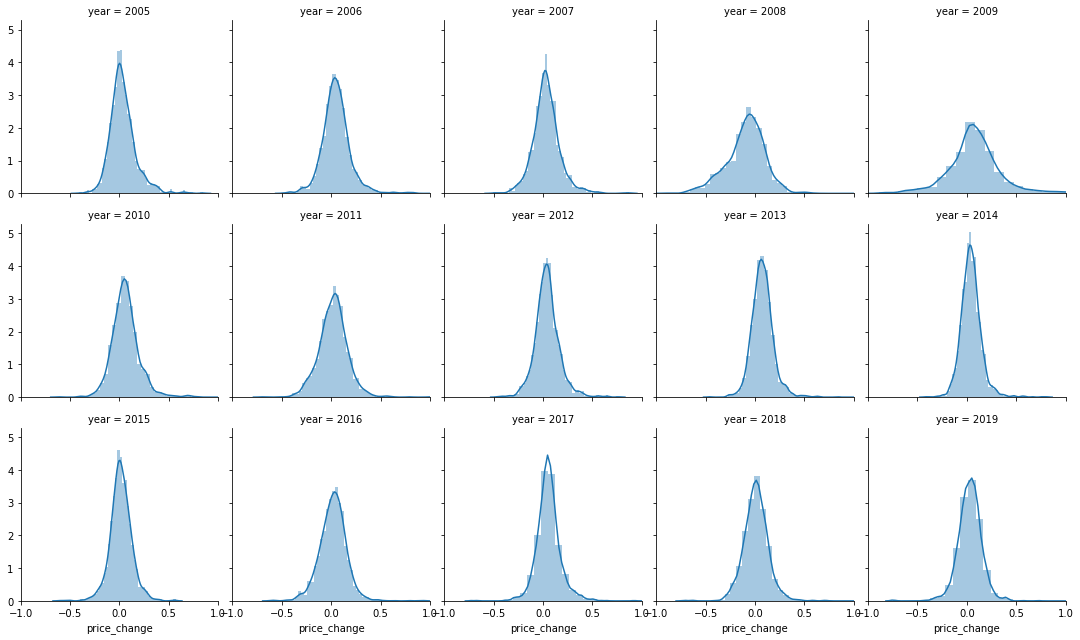We can also take a look at the price trends for individual stocks, or a group of stocks as shown below. To do this we must groupby ticker once again, and take a cumulative sum of the price changes for the given ticker. Now that we have the cumulative returns stored in a new column, we can plot them across dates easily. I encourage you to change ticker_subset to look at different companies' performance.

In :
ticker_subset =  ['FB', 'AMZN', 'NFLX', 'GOOGL', 'AAPL']
df = fund[['date', 'ticker', 'price_change']].copy()  # make a copy for plotting
df = df[df['ticker'].isin(ticker_subset)]  # select only interested tickers
df['returns'] = df.groupby('ticker')['price_change'].cumsum()  # calculate cumulative returns

plt.rcParams['figure.figsize'] = [12, 6]  # adjust plot size
sns.lineplot(data=df, x='date', y='returns', hue='ticker')
plt.show()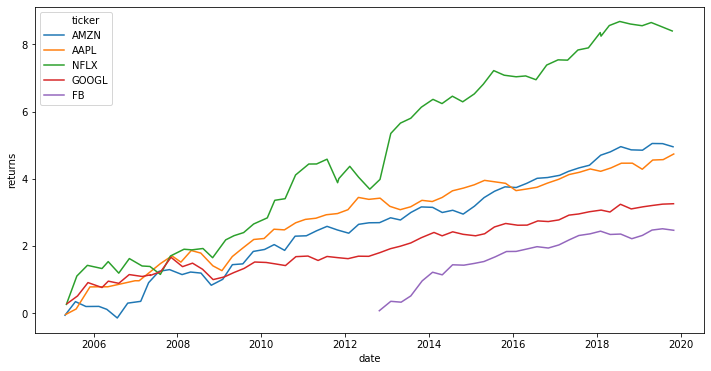Because our model is only concerned with whether the price moved up or down and not by how much, we should take a look at how our movement variable changes over time. To do this we have to groupby year and then calculate the proportion of up movements in each year. Since total movements is given by x.shape we can just sum all the occurrences of up and then divide.

In the plot we can see that between 2005 and 2020, the proportion of up movements fluctuates between 50 and 75 percent, with the exception of 2008 which only experienced about 30% up movements.

In :
yearly_movement = fund.groupby('year')['movement'].apply(lambda x: (x == 'up').sum() / x.shape)
yearly_movement.plot()

Out:
<matplotlib.axes._subplots.AxesSubplot at 0x19ddb5f22e0>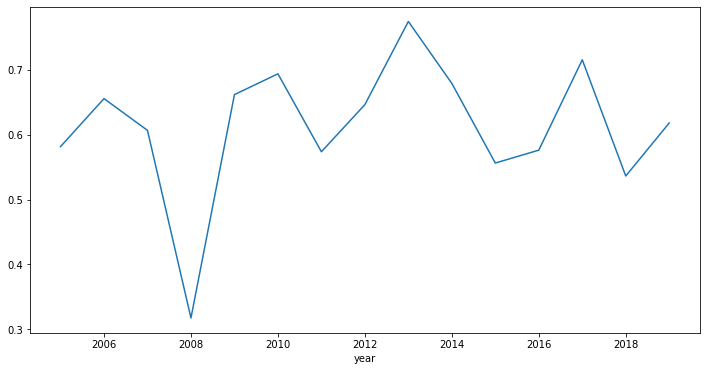We can take a more detailed look at this by plotting the individual price changes across time. Since this results in a lot of data points, I take a 10% sample from the original dataset to use for plotting. Furthermore, we can create a cut function to label the market capitalizations (marketcap) of each data point into three equal groups: small cap, medium cap, and large cap.

The plot supports what we concluded previously. The distribution appears to be normal, centered slightly above 0. We can also see the drawdown from 2008-2010 in a more detailed way, as many companies experience negative price changes.

In :
def cut(x, bins, labels=None):
"""Splits x into [bins] equal groups"""
percentiles = np.arange(0, 100, 100 / bins)
cutoffs = np.nanpercentile(x, percentiles) # value of each percentile in x
out = np.digitize(x, cutoffs) # value of each group the respective x value falls in
if labels:  # convert bins to label names if labels are given
lab_dict = dict(zip(range(1, bins+1), labels))
return pd.Series(out).replace(lab_dict).values
else:
return out

fund['mktcap_bin'] = cut(fund['marketcap'], 3, ['small cap', 'medium cap', 'large cap'])
plot_data = fund.sample(fund.shape // 10, random_state=SEED)  # select 10% of data points
sns.scatterplot(x='date', y='price_change', hue="mktcap_bin", alpha=.5,
data=plot_data, hue_order=['small cap', 'medium cap', 'large cap'])
del plot_data  # no longer needed, clear from memory

plt.xlim(start_date, end_date)
plt.ylim(-1, 1)
plt.show()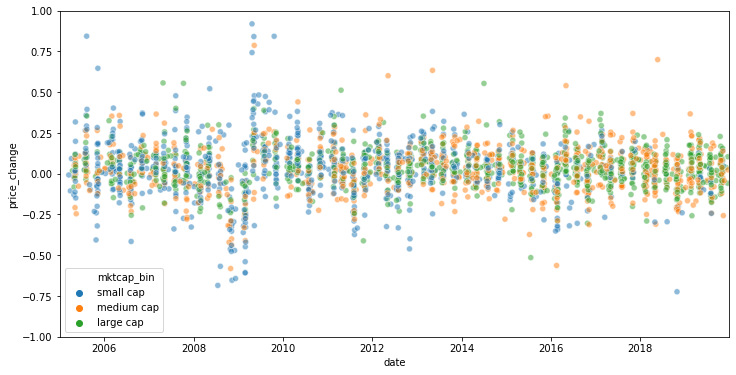Now that we assigned a bin for the marketcap of each stock, lets take a closer look at how the price_change distribution varies between each group. We can do this by creating violin plots for each marketcap bin where the y-axis is the price_change. This graph gives shows us that while the mean price_change is about the same for each group, the spread decreases as marketcap increases. This makes sense because we expect larger companies to have lower volatility. This can be attributed to larger trading volumes, more clear news from large companies, or many other factors.

In :
# sort by mktcap_bin so the x-axis is in order: small, medium, large
sns.violinplot(data=fund.sort_values('mktcap_bin', ascending=False), inner='quartile', x='mktcap_bin', y='price_change')
plt.ylim(-1, 1)
plt.show()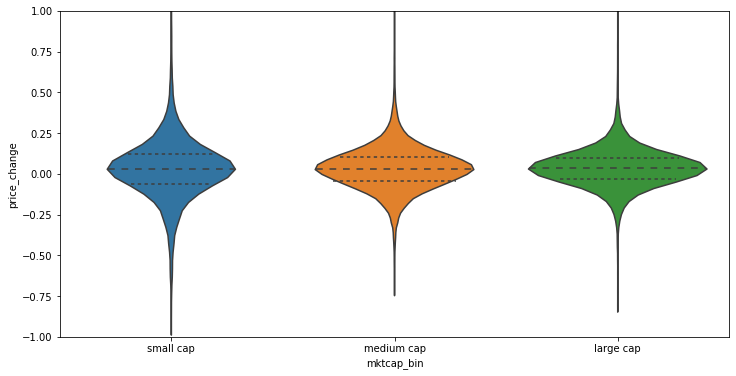Lastly, we can gain some idea of the relationship between features by constructing a correlation matrix between them. For this example I will just look at the balance sheet attributes, in addition to the price. Still focusing on change, we calculate the pct_change for each attribute grouping first by ticker and then selecting the relevant attributes, attrs. Then we call pandas corr() method to create a correlation matrix and use plt.matshow to plot.

This matrix is not very telling, but begins to give us an idea how the different features are related. We are most concerned with the correlations with price, as seen in the last row or column. There are not any strong correlations, so perhaps combining some of these features with machine learning will be more useful. We do see stronger correlations where we would expect so, between the different asset and liability classes, which we should expect to trend together.

In :
attrs = BS + ['price']
correlations = fund.groupby('ticker')[attrs].pct_change().corr()

plt.rcParams['figure.figsize'] = [15, 15]
plt.matshow(correlations)
plt.xticks(range(correlations.shape), correlations.columns, rotation=90, fontsize=12)
plt.yticks(range(correlations.shape), correlations.columns, fontsize=12)
cb = plt.colorbar()
cb.ax.tick_params(labelsize=14)
plt.show()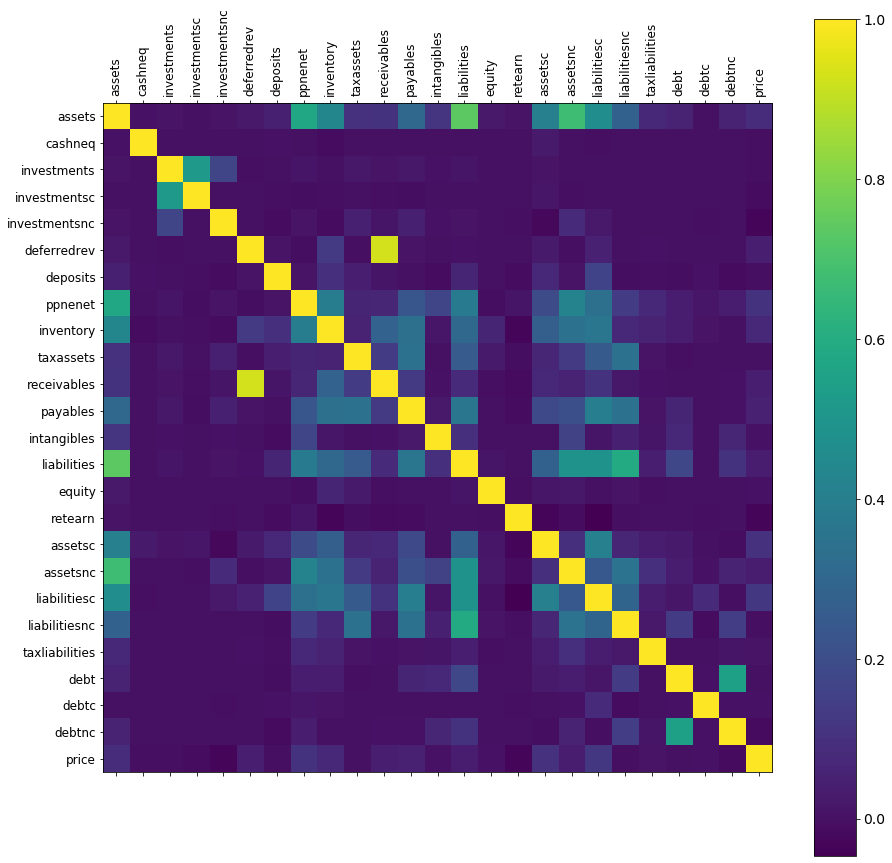# Hypothesis Testing¶

From our previous exploratory data analysis (EDA), we found that there may be a relationship between the standard deviation of price changes for a company, and that company's marketcap. We can test this hypothesis using a linear fit against the relevant data. First we summarize each ticker, taking the mean marketcap for each, and standard deviation of price changes. We should also take the log of the marketcaps for each ticker to even out the distribution, since there are many small companies, with only a few very large ones.

In :
ticker_group = fund.groupby('ticker')
ticker_data = pd.DataFrame(index=fund['ticker'].unique())  # create new dataframe to hold data for each ticker
ticker_data['ticker'] = ticker_data.index
ticker_data['std'] = ticker_group['price_change'].std()
ticker_data['marketcap'] = ticker_group['marketcap'].mean()
ticker_data['log_mktcap'] = np.log(ticker_data['marketcap'].values)
ticker_data.dropna(inplace=True)


We can plot this relationship using seaborn's lmplot which shows that there is a slight negative relationship between these factors.

In :
sns.lmplot(data=ticker_data, x='log_mktcap', y='std', aspect=2, scatter_kws={'alpha': .25})
plt.ylim(0, .5)
plt.show()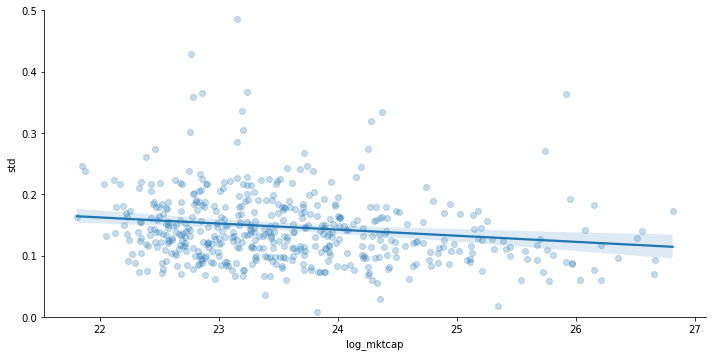We can also use statsmodels to see the statistics of this fit. While the R-squared value is poor, only accounting for 1.8% of the variance, the $\mathrm{p-value} = .003 \leq \alpha = .05$ which means there is a statistically significant relationship between the (log) marketcap of a company, and it's share price's volatility.

In :
import statsmodels.formula.api as smf
model = smf.ols('std~log_mktcap', data=ticker_data)
results = model.fit()
results.summary()

Out:
Dep. Variable: R-squared: std 0.018 OLS 0.016 Least Squares 9.124 Sun, 17 May 2020 0.00265 21:00:25 602.28 495 -1201. 493 -1192. 1 nonrobust
coef std err t P>|t| [0.025 0.975] 0.3813 0.078 4.902 0.000 0.228 0.534 -0.0100 0.003 -3.021 0.003 -0.016 -0.003
 Omnibus: Durbin-Watson: 436.241 2.047 0 14439.3 3.688 0 28.41 570

Warnings:
 Standard Errors assume that the covariance matrix of the errors is correctly specified.

# Machine Learning¶

Now that we have done some basic hypothesis testing, we can move into more advanced machine learning techniques. To do this we must first convert the movement label to a binary [1, 0] score so it can be used in our models. As previously mentioned, we should split the data at 2017/01/01 for our training set and out-of-sample (oos) testing set.

Since we want to predict future price movement, we shift movement column up 1 so it refers to the next change in share price.

In :
data = fund.copy()
data['movement'] = data.groupby('ticker')['movement'].shift(-1)  # movement now associated with future movement
data['mvmt_binary'] = np.where(data['movement'] == 'up', 1, 0)

# Split training and testing set at January 1st, 2017
predictor_df = data[data['date'] < datetime.datetime(year=2017, month=1, day=1)]
oos_df = data[data['date'] >= datetime.datetime(year=2017, month=1, day=1)]

# Split into X and Y and convert to numpy matrices
X = predictor_df[features].values
y = predictor_df['mvmt_binary'].values

X_oos = oos_df[features].values
y_oos = oos_df['mvmt_binary'].values


We will be looking at the prediction performance between a logistic regression and random forest, so first import all the necessary modules from sklearn.

In :
from sklearn.linear_model import LogisticRegression
from sklearn.ensemble import RandomForestClassifier
from sklearn.impute import SimpleImputer
from sklearn.model_selection import GridSearchCV, StratifiedKFold
from sklearn.neighbors import KNeighborsClassifier
from sklearn.metrics import auc


For the logistic regression we need to adjust the max iterations to be the length of the dataset to ensure the model converges. For the random forest I will be using 250 decision trees with a max depth of 10 and also require at least 10 samples to split at a decision node.

Since the data is not perfectly clean, we will use sklearn's SimpleImputer to fill NaN values with the mean of the values for that feature. We then use fit_transform to clean our data sets and then fit our models using the training data. Once the models are fitted, we use predict_proba to test our out-of-sample data against the model.

In :
# Create classification models
log_reg = LogisticRegression(max_iter=X.shape)
rf = RandomForestClassifier(n_estimators=250, max_depth=10,
min_samples_split=10, max_features="auto")
imp = SimpleImputer(missing_values=np.nan, strategy='mean')  # object for cleaning data

# Clean training and testing sets
train_clean = imp.fit_transform(X)
test_clean = imp.fit_transform(X_oos)

# Fit models
log_reg.fit(train_clean, y)
rf.fit(train_clean, y)

# Get scores of models
log_scores = log_reg.predict_proba(test_clean)[:,1]
rf_scores = rf.predict_proba(test_clean)[:,1]


We can look at the coefficients from the logistic regression and the feature importances from the random forest to understand how the different models are weighting the various features.

In :
def pretty_dict(val, per_row=5):
"""Prints dict items to stdout where per_row refers
to the number of items printed on each line"""
out = ""
for i, (k, v) in enumerate(val.items(), 1):
out += f"'{k}': {v}, "
if i % per_row == 0:
out += '\n'
return out

# Create dict of features' coefficients, sort, and format for printing
log_coefs = dict(zip(features, log_reg.coef_))
log_coefs = sorted(log_coefs.items(), key=lambda item: item, reverse=True)
log_coefs = {k: '{:.3e}'.format(v) for k, v in log_coefs}

# Create dict of features' importances, sort, and format for printing
rf_importances = dict(zip(features, rf.feature_importances_))
rf_importances = sorted(rf_importances.items(), key=lambda item: item, reverse=True)
rf_importances = {k: round(v, 3) for k, v in rf_importances}

print(f"Logistic Regression Coefficients:\n{pretty_dict(log_coefs, 4)}")
print(f"Random Forest Feature Importances:\n{pretty_dict(rf_importances, 5)}")

Logistic Regression Coefficients:
'intexp': 6.370e-10, 'ebit': 1.883e-10, 'sbcomp': 1.855e-10, 'workingcapital': 1.236e-10,
'liabilitiesc': 1.204e-10, 'ncfdiv': 6.492e-11, 'ncfcommon': 5.959e-11, 'cashneq': 3.982e-11,
'debtnc': 3.754e-11, 'ev': 3.641e-11, 'ncfbus': 3.098e-11, 'capex': 2.693e-11,
'equity': 2.534e-11, 'opex': 2.358e-11, 'ncfi': 1.850e-11, 'ncff': 1.731e-11,
'fcf': 1.529e-11, 'gp': 1.366e-11, 'ncfx': 1.259e-11, 'liabilities': 1.236e-11,
'assetsnc': 3.877e-12, 'retearn': 3.119e-12, 'revenue': 2.090e-12, 'investments': 1.618e-12,
'payables': 1.255e-12, 'bvps': 6.074e-16, 'pb': 5.001e-16, 'pe': 4.702e-16,
'sps': 3.455e-16, 'ps': 3.033e-16, 'de': 2.065e-16, 'currentratio': 1.077e-16,
'fcfps': 3.636e-17, 'eps': 3.045e-17, 'grossmargin': 2.812e-17, 'ebitdamargin': 2.353e-17,
'netmargin': 1.702e-17, 'divyield': 9.849e-19, 'payoutratio': -5.042e-18, 'evebit': -4.036e-16,
'evebitda': -6.696e-16, 'deposits': -1.257e-12, 'receivables': -5.123e-12, 'liabilitiesnc': -5.313e-12,
'taxassets': -5.866e-12, 'taxliabilities': -6.571e-12, 'investmentsnc': -7.861e-12, 'intangibles': -8.103e-12,
'ppnenet': -9.757e-12, 'opinc': -9.920e-12, 'inventory': -1.024e-11, 'cor': -1.157e-11,
'ncfo': -1.163e-11, 'deferredrev': -1.192e-11, 'ncf': -1.220e-11, 'assets': -1.390e-11,
'ncfdebt': -1.534e-11, 'ncfinv': -1.592e-11, 'sgna': -2.477e-11, 'investmentsc': -2.971e-11,
'debtc': -3.396e-11, 'marketcap': -3.713e-11, 'debt': -3.835e-11, 'depamor': -8.218e-11,
'assetsc': -9.099e-11, 'netinc': -2.060e-10, 'rnd': -2.488e-10, 'taxexp': -2.604e-10,

Random Forest Feature Importances:
'ev': 0.026, 'ncfo': 0.024, 'marketcap': 0.024, 'ncfcommon': 0.024, 'fcfps': 0.022,
'fcf': 0.021, 'ncf': 0.021, 'pb': 0.02, 'pe': 0.02, 'ncfdebt': 0.019,
'evebitda': 0.019, 'ps': 0.019, 'ncfinv': 0.019, 'ncff': 0.018, 'cashneq': 0.018,
'equity': 0.018, 'bvps': 0.018, 'sbcomp': 0.017, 'sps': 0.017, 'capex': 0.017,
'retearn': 0.016, 'ncfi': 0.016, 'gp': 0.016, 'eps': 0.016, 'ebitdamargin': 0.015,
'grossmargin': 0.015, 'revenue': 0.015, 'taxexp': 0.015, 'payables': 0.015, 'de': 0.015,
'netinc': 0.015, 'netmargin': 0.015, 'ncfbus': 0.015, 'assets': 0.015, 'opex': 0.014,
'debt': 0.014, 'ebit': 0.014, 'sgna': 0.014, 'ncfx': 0.014, 'payoutratio': 0.014,
'opinc': 0.014, 'receivables': 0.014, 'depamor': 0.013, 'intangibles': 0.013, 'intexp': 0.013,
'ppnenet': 0.013, 'cor': 0.013, 'assetsc': 0.013, 'liabilities': 0.013, 'evebit': 0.012,
'currentratio': 0.012, 'divyield': 0.012, 'assetsnc': 0.012, 'liabilitiesnc': 0.012, 'workingcapital': 0.012,
'liabilitiesc': 0.011, 'ncfdiv': 0.011, 'investments': 0.01, 'debtc': 0.01, 'debtnc': 0.01,
'inventory': 0.01, 'taxassets': 0.01, 'taxliabilities': 0.01, 'investmentsc': 0.007, 'investmentsnc': 0.007,
'deferredrev': 0.006, 'rnd': 0.006, 'deposits': 0.004,


We can also export one of the decision trees from the random forest to an image to see the actual decision path of one of the trees in the model.

In :
from sklearn.tree import export_graphviz
from IPython.display import Image
import pydot

estimator = rf.estimators_
# Export decision tree as dot file
export_graphviz(estimator, out_file='tree.dot',
feature_names = features,
class_names = None,
rounded = True, proportion = False,
precision = 2, filled = True,
max_depth=3)

# Use pydot to convert dot file to png
(graph,) = pydot.graph_from_dot_file('tree.dot')
graph.write_png('tree.png')
Image(filename='tree.png')  # display image

Out: